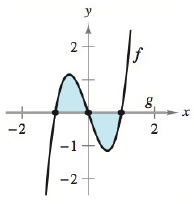Chapter 5.5, Problem 7E### Calculus: An Applied Approach (Min...

10th Edition
Ron Larson
ISBN: 9781305860919

#### Solutions

Chapter
Section### Calculus: An Applied Approach (Min...

10th Edition
Ron Larson
ISBN: 9781305860919
Textbook Problem
1 views

# Finding the Area Bounded by Two Graphs In Exercises 1-8, find the area of the region. See Examples 1, 2, 3, and 4. f ( x ) = 3 ( x 3 − 1 ) g ( x ) = 0To determine

To calculate: The area bounded by the function f(x)=3(x3x) and g(x)=0.

Explanation

Given Information:

The provided function is f(x)=3(x3x) and g(x)=0 and the provided graph is shown below,

Formula used:

The function f(x) and g(x) are the continuous function on interval [a,b] and g(x)f(x) for all x in [a,b], then, the area bounded by the graphs of f(x) and g(x),x=a and x=b is,

A=ab[f(x)g(x)]dx

Calculation:

Consider the provided function is f(x)=3(x3x) and g(x)=0.

In the provided function f(x)=3(x3x) and g(x)=0 where, f(x)g(x).

Now, from the provided graph, the limit of the function is x=1 and x=1.

In the provided graph g(x)f(x)

Now, the area bounded the curve f(x)=3(x3x) and g(x)=0 from point x=1 and x=1

### Still sussing out bartleby?

Check out a sample textbook solution.

See a sample solution

#### The Solution to Your Study Problems

Bartleby provides explanations to thousands of textbook problems written by our experts, many with advanced degrees!

Get Started

#### Find more solutions based on key concepts